#### Thank you for registering.

One of our academic counsellors will contact you within 1 working day.

Please check your email for login details.

Click to Chat

1800-1023-196

+91-120-4616500

CART 0

• 0
MY CART (5)

Use Coupon: CART20 and get 20% off on all online Study Material

ITEM
DETAILS
MRP
DISCOUNT
FINAL PRICE
Total Price: Rs.

There are no items in this cart.
Continue Shopping• Complete JEE Main/Advanced Course and Test Series
• Offered Price: Rs. 15,900
• View Details

# Chapter 4: Triangles Exercise – 4.7

### Question: 1

If the sides of a triangle are 3 cm, 4 cm, and 6 cm long, determine whether the triangle is a right-angled triangle.

### Solution:

We have,

Sides of triangle

AB = 3 cm

BC = 4 cm

AC = 6 cm

∴  AB2 = 32 = 9

BC2 = 42 = 16

AC2 = 62 = 36

Since, AB2 + BC2 ≠ AC2

Then, by converse of Pythagoras theorem, triangle is not a right triangle.

### Question: 2

The sides of certain triangles are given below. Determine which of them right triangles are.

(i) a = 7 cm, b = 24 cm and c = 25 cm

(ii) a = 9 cm, b = 16 cm and c = 18 cm

(iii) a = 1.6 cm, b = 3.8 cm and c = 4 cm

(iv) a = 8 cm, b = 10 cm and c = 6 cm

### Solution:

We have,

a = 7 cm, b = 24 cm and c = 25 cm

∴ a2 = 49, b2 = 576 and c2 = 625

Since, a2 + b2 = 49 + 576 = 625 = C2

Then, by converse of Pythagoras theorem, given triangle is a right triangle.

We have,

a = 9 cm, b = 16 cm and c = 18 cm

∴ a2 = 81, b2 = 256 and c2 = 324

Since, a2 + b2 = 81 + 256 = 337 ≠ c2

Then, by converse of Pythagoras theorem, given triangle is not a right triangle.

We have,

a = 1.6 cm, b = 3.8 cm and C = 4 cm

∴ a2 = 64, b2 = 100 and c2 = 36

Since, a2 + c2 = 64 + 36 = 100 = b2

Then, by converse of Pythagoras theorem, given triangle is a light triangle.

### Question: 3

A man goes 15 metres due west and then 8 metres due north. How far is lie from the starting point?

### Solution: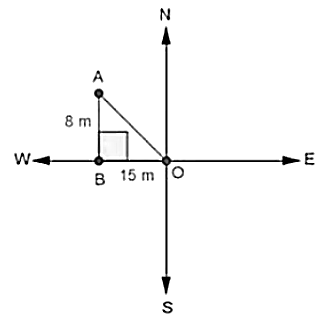Let the starting point of the man be O and final point be A.

∴ In ∆ABO, by Pythagoras theorem AO2 = AB2 + BO2

⟹ AO2 = 82 + 152

⟹ AO2 = 64 + 225 = 289

⟹ AO =  √289 = 17m

∴  He is 17m far from the starting point.

### Question: 4

A ladder 17 m long reaches a window of a building 15 m above the ground. Find the distance of the foot of the ladder from the building.

### Solution:In ∆ABC, by Pythagoras theorem

AB2 + BC2 = AC2

⟹ 152 + BC2 = 172

⟹ 225 + BC2 = 172

⟹ BC2 = 289 – 225

⟹ BC2 = 64

⟹ BC = 8 m

∴ Distance of the foot of the ladder from building = 8 m

### Question: 5

Two poles of heights 6 m and 11 m stand on a plane ground. If the distance between their feet is 12 m, find the distance between their tops.

### Solution:

Let CD and AB be the poles of height 11 and 6 in.Therefore CP = 11 - 6 = 5 m

From the figure we may observe that AP = 12m

In triangle APC, by applying Pythagoras theorem

Ap2 + PC2 = AC2

122 + 52 = AC2

AC2 = 144 + 25 = 169

AC = 13

Therefore distance between their tops = 13 m.

### Question: 6

In an isosceles triangle ABC. AB = AC = 25 cm, BC = 14 cm. Calculate the altitude from A on BC.

### Solution:

We have

AB = AC = 25 cm and BC = 14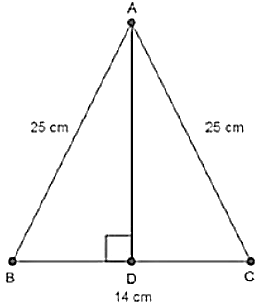In ∆ABD and ∆ACD

∠ADB = ∠ADC                   [Each = 90°]

AB = AC                                [Each = 25 cm]

AD = AD                               [Common]

Then, ∆ABD ≅ ∆ACD      [By RHS condition]

∴ BD = CD = 7 cm              [By c.p.c.t]

In ∆ADB, by Pythagoras theorem

AD2 + BD2 = AB2

⟹ AD2 + 72 = 252

⟹ AD2 = 625 – 49 = 576

⟹ AD = √576 = 24 cm

### Question: 7

The foot of a ladder is 6 m away from a wall and its top reaches a window 8 m above the ground. If the ladder is shifted in such a way that its foot is 8 m away from the wall, to what height does its tip reach?

### Solution: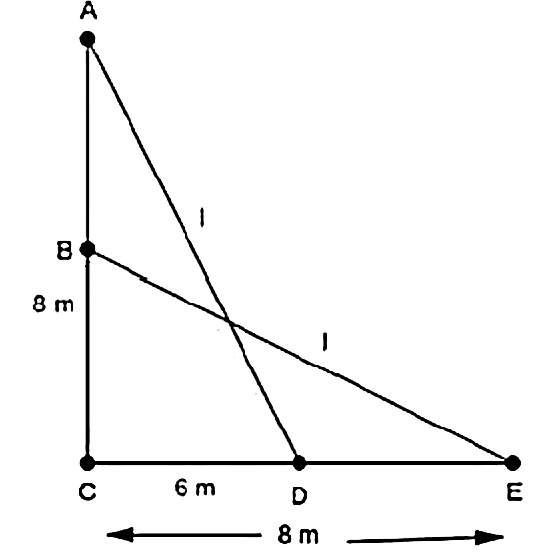Let length of ladder be AD = BE = l m

In ∆ACD, by Pythagoras theorem

AD2 = AC2 + CD2

⟹ l2 = 82 + 62 ... (i)

In ∆BCE, by pythagoras theorem

BE2 = BC2 + CE2

⟹ l2 = BC2 + 82 ... (ii)

Compare (i) and (ii)

BC2 + 82 = 82 + 62

⟹ BC2 + 62

⟹ BC = 6 m

### Question: 8

Two poles of height 9 in and 14 m stand on a plane ground. If the distance between their feet is 12 in, find the distance between their tops.

### Solution: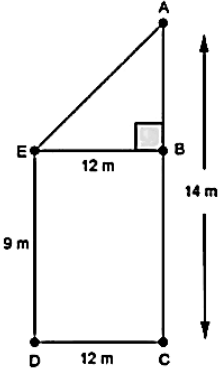We have,

AC = 14 m. DC = 12m and ED = BC = 9 m

Construction: Draw EB ⊥ AC

∴ AB = AC –  BC = 14 — 9 = 5 m

And, EB = DC = 12m

In ∆ABE, by Pythagoras theorem,

AE2 = AB2 + BE2

⟹ AE2 = 52 + 122

⟹ AE2 = 25 + 144 = 169

⟹ AE= √169 = 13 m

∴  Distance between their tops = 13 m

### Question: 9

Using Pythagoras theorem determine the length of AD in terms of b and c shown in Fig. 4.219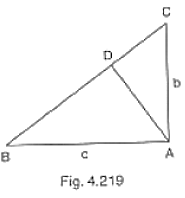### Solution:

We have,

In ∆BAC, by Pythagoras theorem

BC2 = AB2 + AC2

⟹ BC2 = c2 + b2In ∆ABD and ∆CBA

∠B = ∠B                      [Common]

∠ADB = ∠BAC             [Each 90°]

Then, ∆ABD ͏~ ∆CBA  [By AA similarity]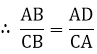[Corresponding parts of similar ∆ are proportional]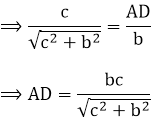### Question: 10

A triangle has sides 5 cm, 12 cm and 13 cm. Find the length to one decimal place, of the perpendicular from the opposite vertex to the side whose length is 13 cm.

### Solution:Let, AB = 5cm, BC = 12 cm and AC = 13 cm. Then, AC2 = AB2 + BC2. This proves that ∆ABC is a fight triangle. right angles at B. Let BD be the length of perpendicular from B on AC.= 30 cm2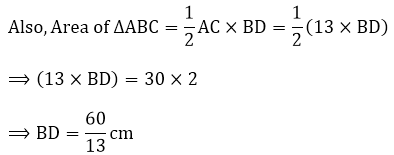## Complete JEE Main/Advanced Course and Test Series

OFFERED PRICE: Rs. 15,900
View Details Xpress Buy

### Course Features

• 731 Video Lectures
• Revision Notes
• Test paper with Video Solution
• Mind Map
• Study Planner
• NCERT Solutions
• Discussion Forum
• Previous Year Papers

### Course Features

• 728 Video Lectures
• Revision Notes
• Previous Year Papers
• Mind Map
• Study Planner
• NCERT Solutions
• Discussion Forum
• Test paper with Video Solution